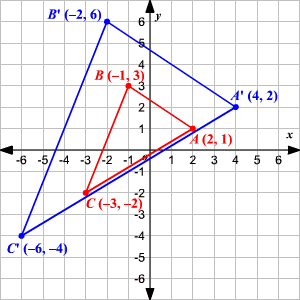# Transformation of Graphs Using Matrices - Dilation

A type of transformation that occurs when a figure is enlarged or reduced, the transformation is called a dilation . A dilation requires a center point and a scale factor . You can use scalar multiplication to perform dilations in matrices.

Example:

Write the vertex matrix for $\Delta ABC$ with $A\left(2,1\right),B\left(-1,3\right),\text{\hspace{0.17em}}\text{\hspace{0.17em}}\text{and}\text{\hspace{0.17em}}\text{\hspace{0.17em}}C\left(-3,-2\right)$ . Then find the coordinates of the vertices of $\Delta {A}^{\text{'}}{B}^{\text{'}}{C}^{\text{'}}$ after a dilation by a scale factor of $2$ .

$\left[\begin{array}{ccc}\hfill 2& \hfill -1& \hfill -3\\ \hfill 1& \hfill 3& \hfill -2\end{array}\right]$

The scale factor is $2$ . So, multiply the vertex matrix by the scale factor of $2$ .

$2\left[\begin{array}{ccc}\hfill 2& \hfill -1& \hfill -3\\ \hfill 1& \hfill 3& \hfill -2\end{array}\right]=\left[\begin{array}{ccc}\hfill 4& \hfill -2& \hfill -6\\ \hfill 2& \hfill 6& \hfill -4\end{array}\right]$

The coordinates of the vertices of $\Delta {A}^{\text{'}}{B}^{\text{'}}{C}^{\text{'}}$ are ${A}^{\text{'}}\left(4,2\right),\text{\hspace{0.17em}}\text{\hspace{0.17em}}{B}^{\text{'}}\left(-2,6\right),\text{\hspace{0.17em}}\text{\hspace{0.17em}}\text{and}\text{\hspace{0.17em}}\text{\hspace{0.17em}}{C}^{\text{'}}\left(-6,-4\right)$ .